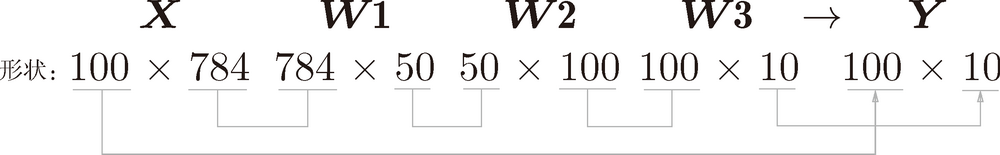# 深度学习入门：基于 Python 的理论与实现 (24)：神经网络 3.6.3(批处理)

>>> x, _ = get_data()
>>> network = init_network()
>>> W1, W2, W3 = network['W1'], network['W2'], network['W3']
>>>
>>> x.shape
(10000, 784)
>>> x.shape
(784,)
>>> W1.shape
(784, 50)
>>> W2.shape
(50, 100)
>>> W3.shape
(100, 10)x, t = get_data()
network = init_network()

batch_size = 100 #  批数量
accuracy_cnt = 0

for i in range(0, len(x), batch_size):
x_batch = x[i:i+batch_size]
y_batch = predict(network, x_batch)
p = np.argmax(y_batch, axis=1)
accuracy_cnt += np.sum(p == t[i:i+batch_size])

>>> list( range(0, 10) )
[0, 1, 2, 3, 4, 5, 6, 7, 8, 9]
>>> list( range(0, 10, 3) )
[0, 3, 6, 9]

1 矩阵的第 0 维是列方向，第 1 维是行方向。——译者注
>>> x = np.array([[0.1, 0.8, 0.1], [0.3, 0.1, 0.6],
...     [0.2, 0.5, 0.3], [0.8, 0.1, 0.1]])
>>> y = np.argmax(x, axis=1)
>>> print(y)
[1 2 1 0]

>>> y = np.array([1, 2, 1, 0])
>>> t = np.array([1, 2, 0, 0])
>>> print(y==t)
[True True False True]
>>> np.sum(y==t)
3